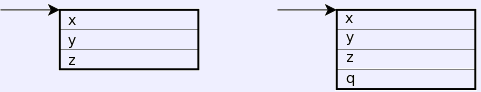Translating InheritanceTranslating derived classes relies on the first part of the dynamic structures being the same. That way a pointer to the derived class object also points to the base class part of that object.class Base {private:    int x,y,z;public:    Base(int a) {        x = a;        y = z = 0;    }    void joe(int m) {        y = m;    }};class Derived: public Base {private:    int q;public:    Derived(int m, int n): Base(m) {        q = n;    }    int bill(int n) {        q += n;        return z;    }};main() {    int t;    Derived d(20, 30);    d.joe(5);    t = d.bill(30);} struct Base_dynamic_part {    int x,y,z;};void Base_Base(struct Base_dynamic_part *this, int a) {    this->x = a;    this->y = this->z = 0;}void Base_joe(struct Base_dynamic_part *this, int m) {    this->y = m;}struct Derived_dynamic_part {    // Exact copy of Base_dynamic_part.    int x,y,z;    // Add new stuff.    int q;};void Derived_Derived(Derived_dynamic_part *this, int m, int n) {    Base_Base((Base_dynamic_part *)this, m);    this->q = n;}int Derived_bill(Derived_dynamic_part *this, int n) {    this->q += n;    return this->z;}main() {    int t;    struct Derived_dynamic_part d;    Derived_Derived(&d, 20, 30);    Base_joe((Base_dynamic_part *)&d, 5);    t = Derived_bill(&d, 30);}